# RD Sharma Solutions for Class 9 Maths Chapter 13 Linear Equations in Two Variables Exercise 13.3

RD Sharma Solutions for Class 9 Maths are given here for Chapter 13 exercise 13.3. In this exercise, students will learn how to solve linear equations with the help of various solved examples. Exercise 13.3 of chapter 13 deals with graph of linear equations in two variables. In order to find the graph of linear equations in two variables, first we need to obtain the linear equation in the form ax + by + c = 0. Express y in terms of x. Given any values of x and calculate the corresponding values of y. Plot the points on the graph and draw a line passing through points marked. The lone so obtained is the graph of the given equation.

## Download PDF of RD Sharma Solutions for Class 9 Maths Chapter 13 Linear Equations in Two Variables Exercise 13.3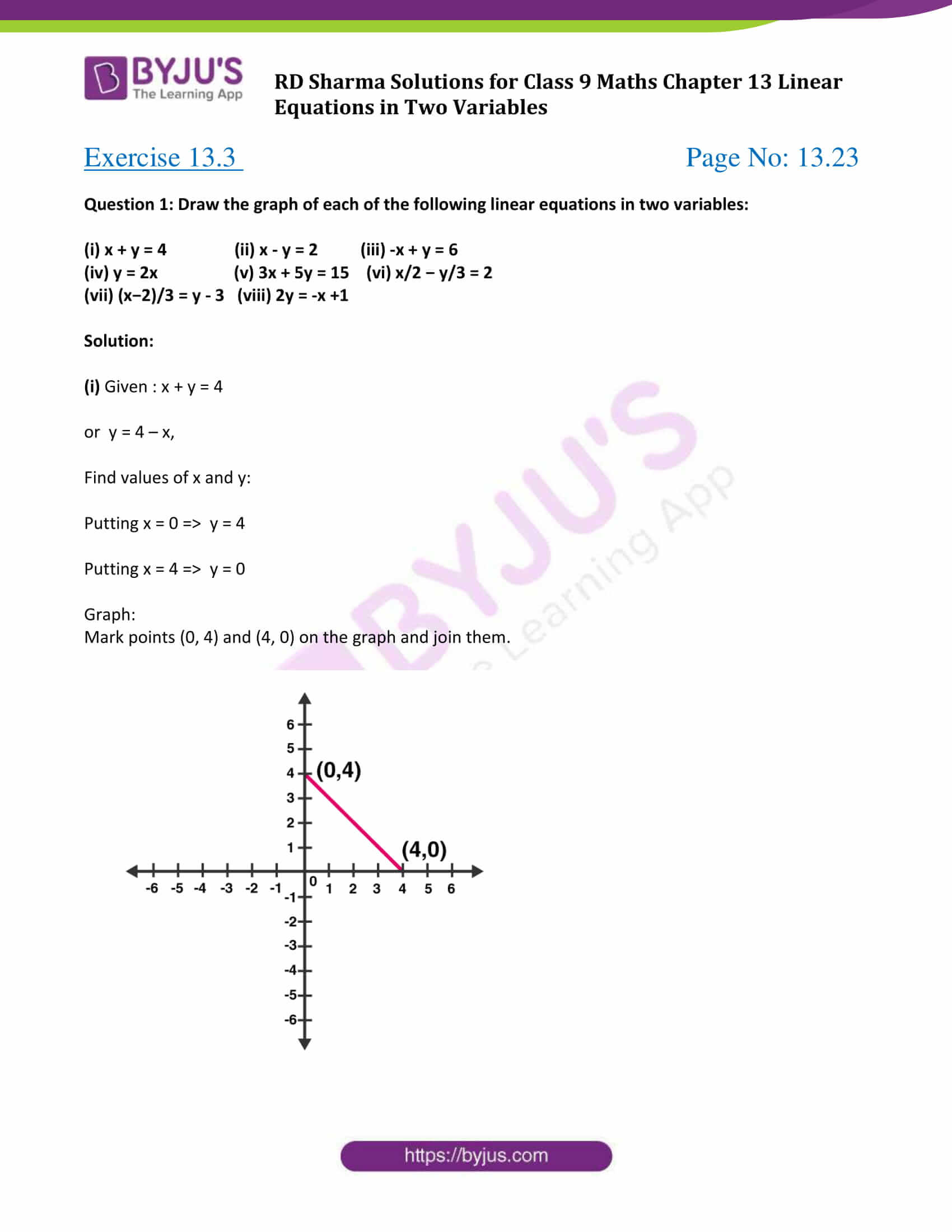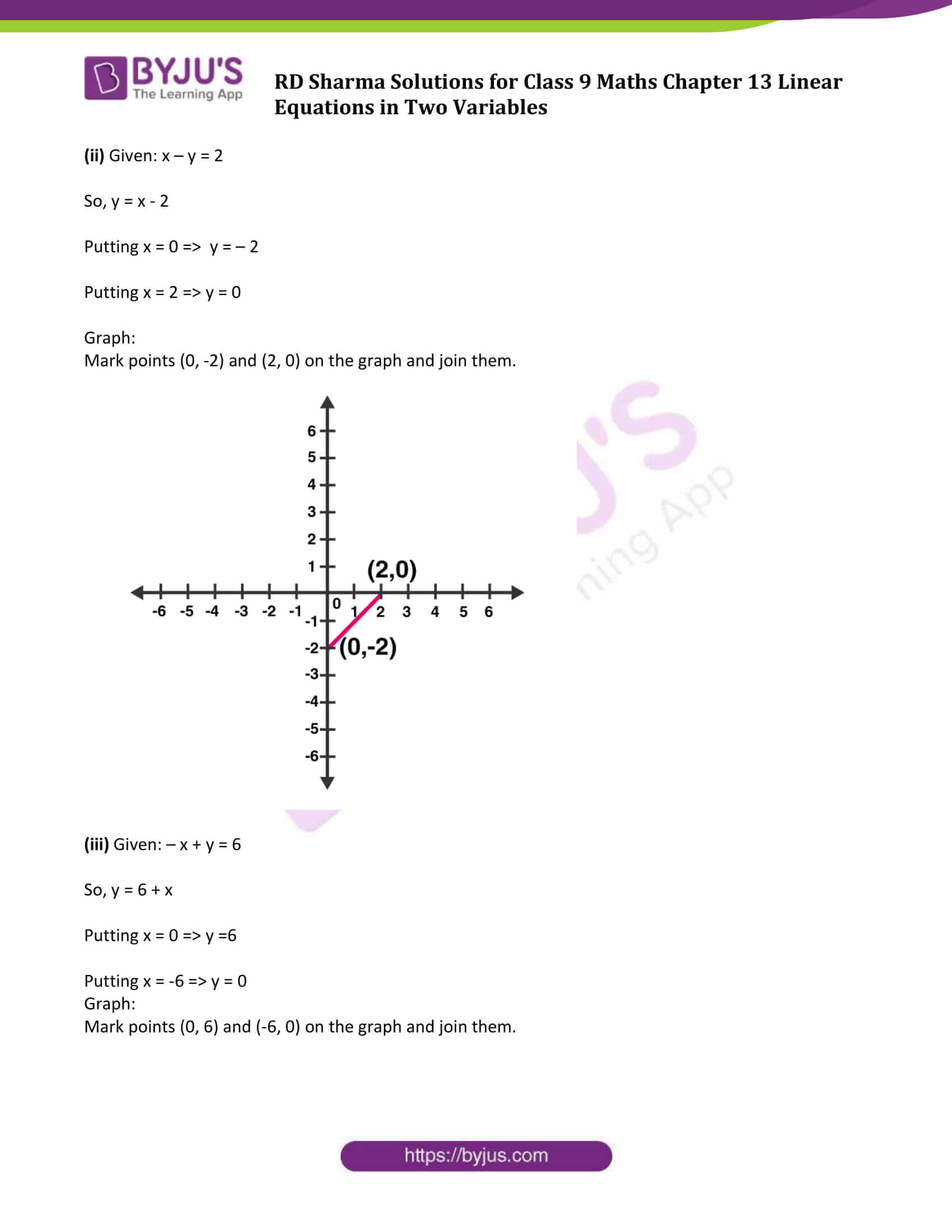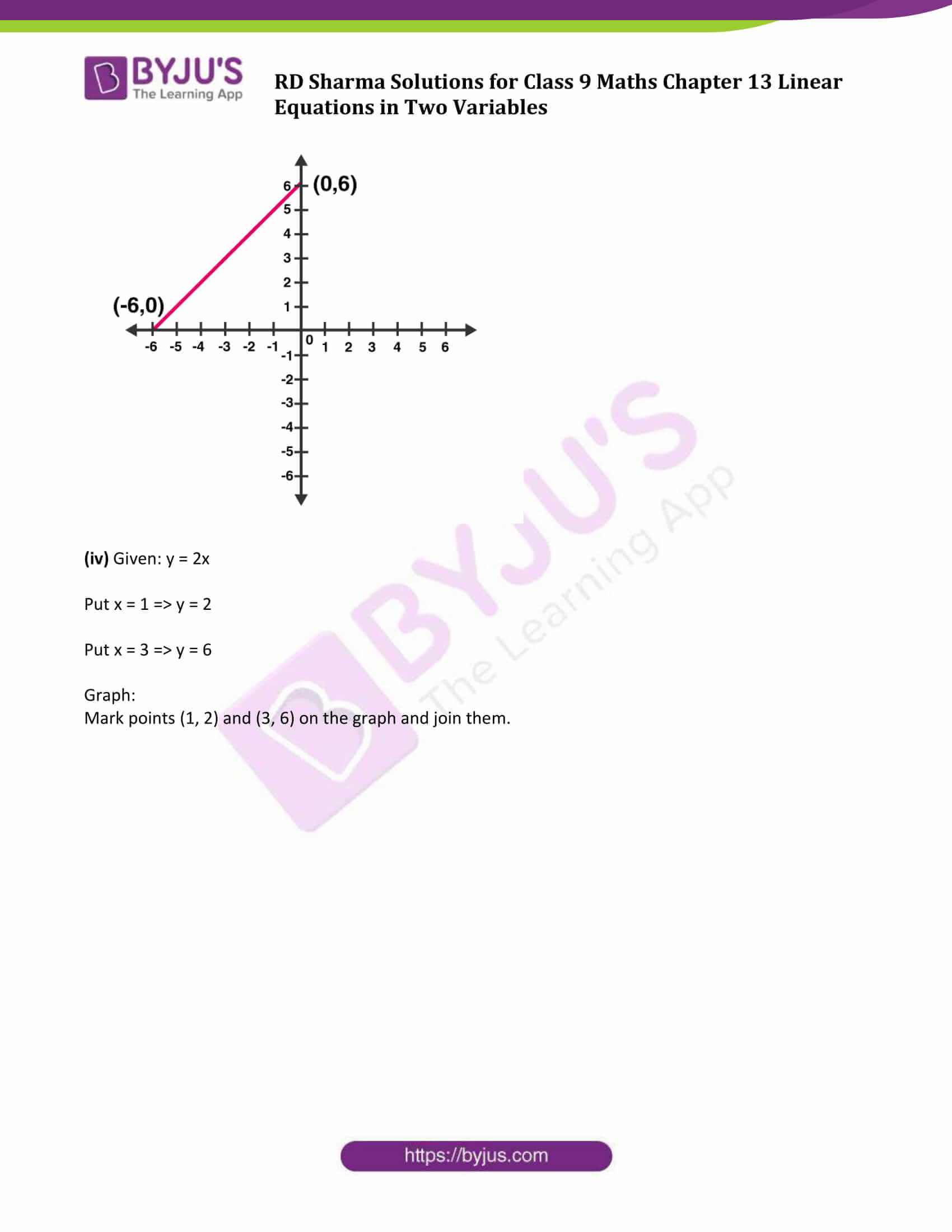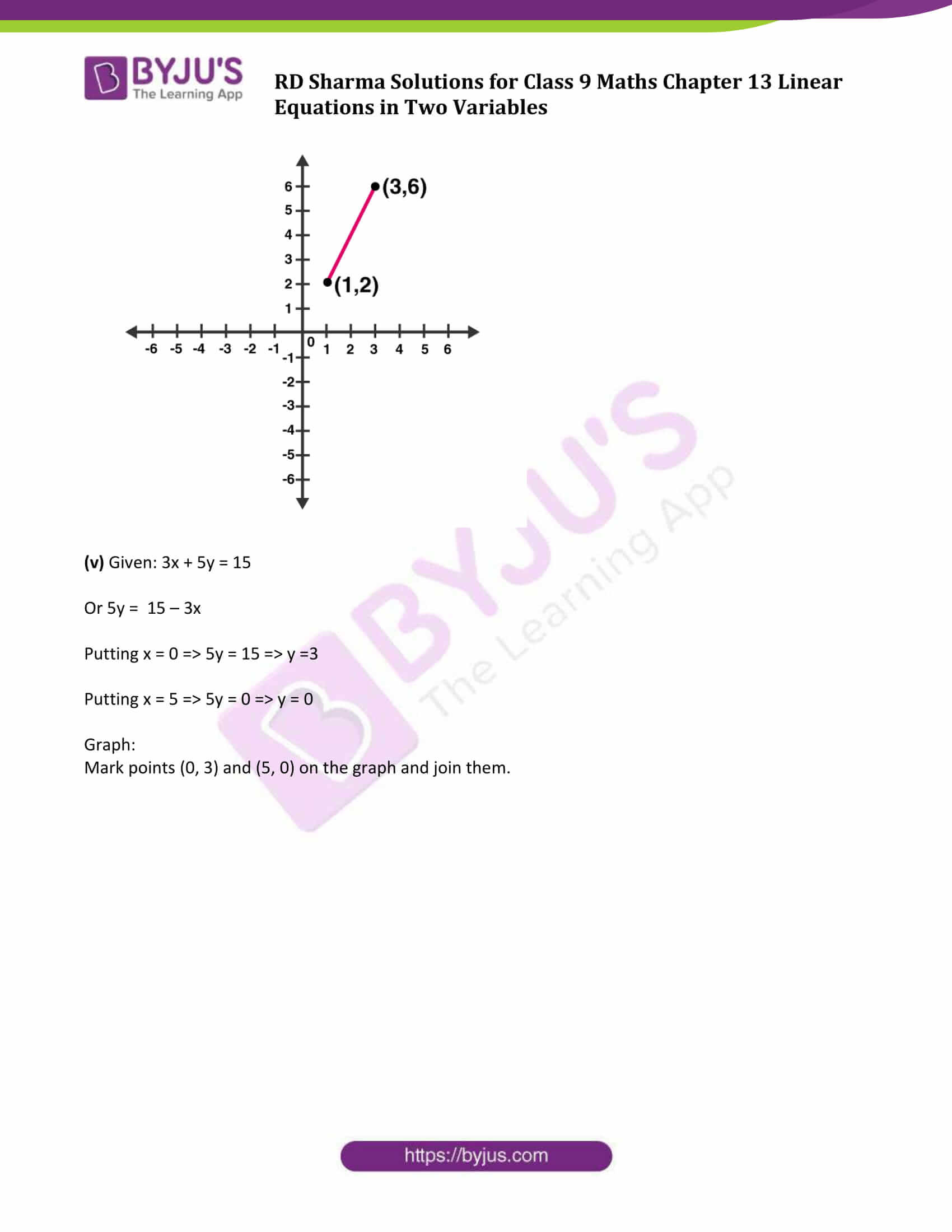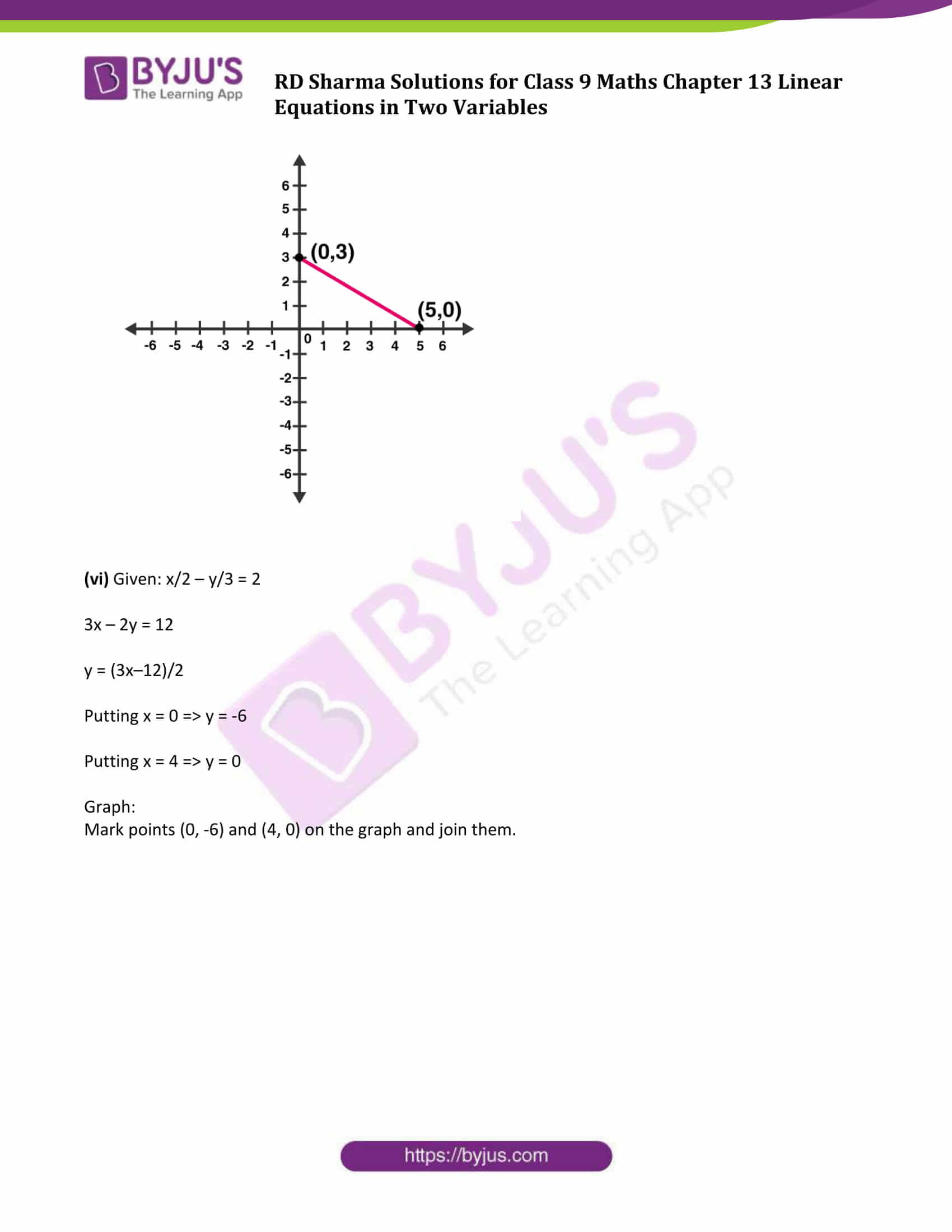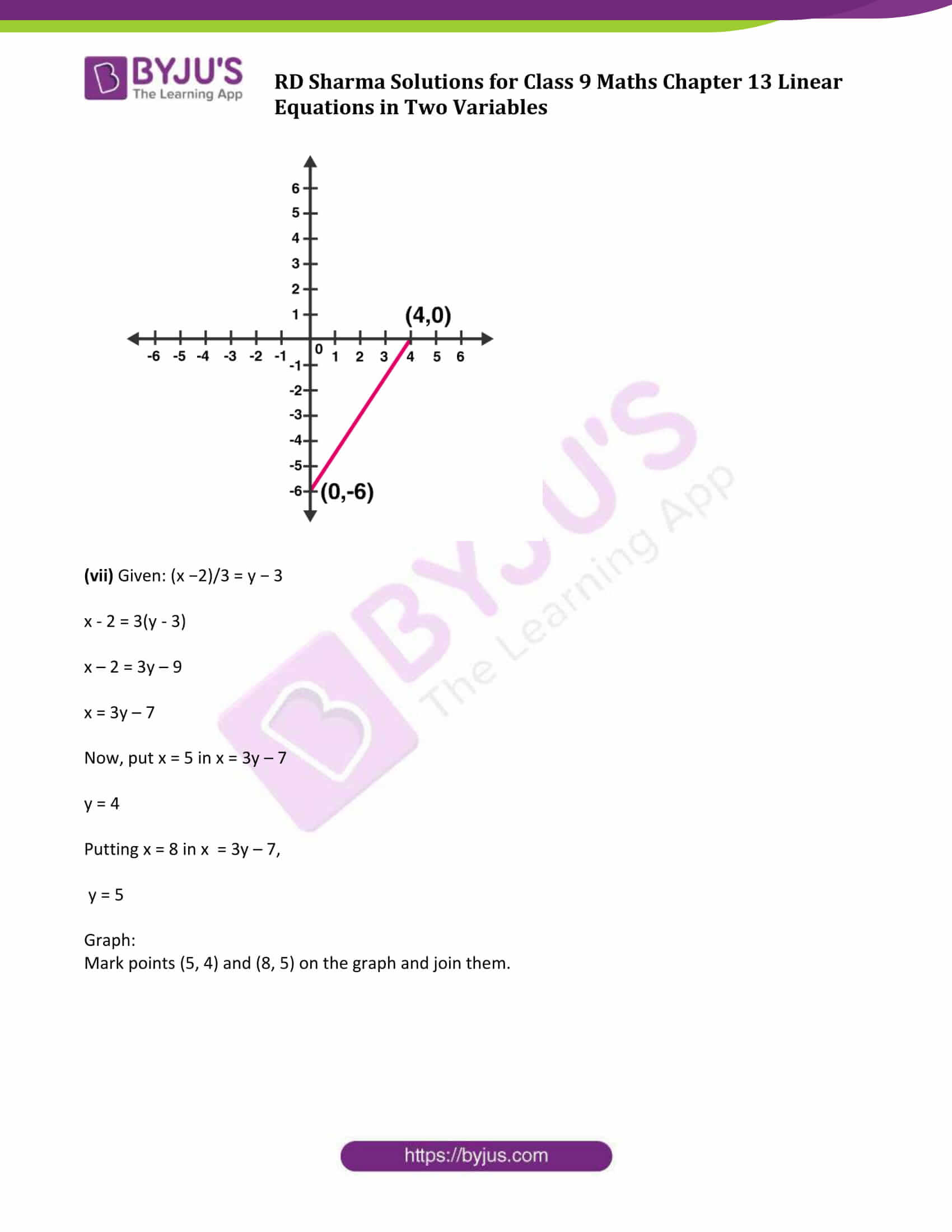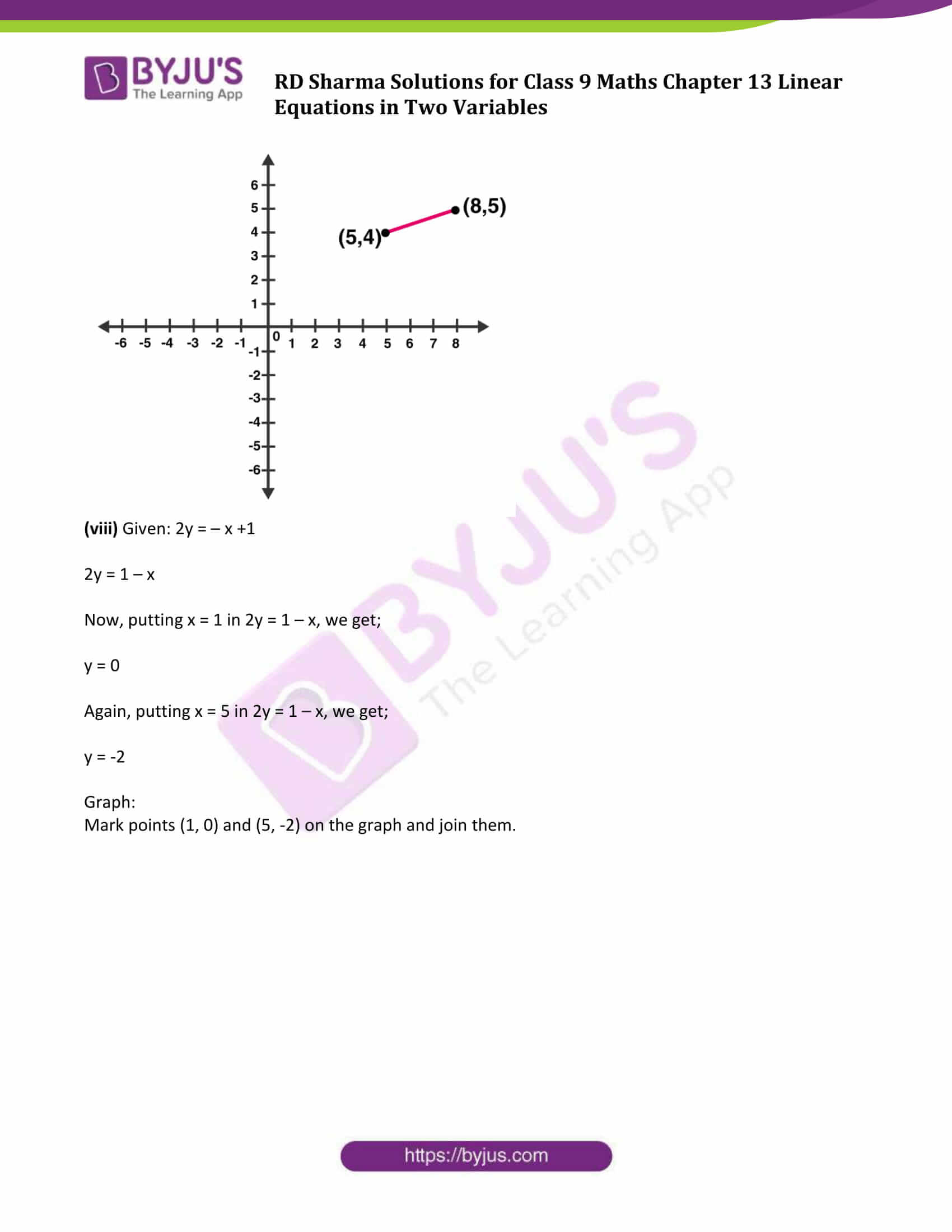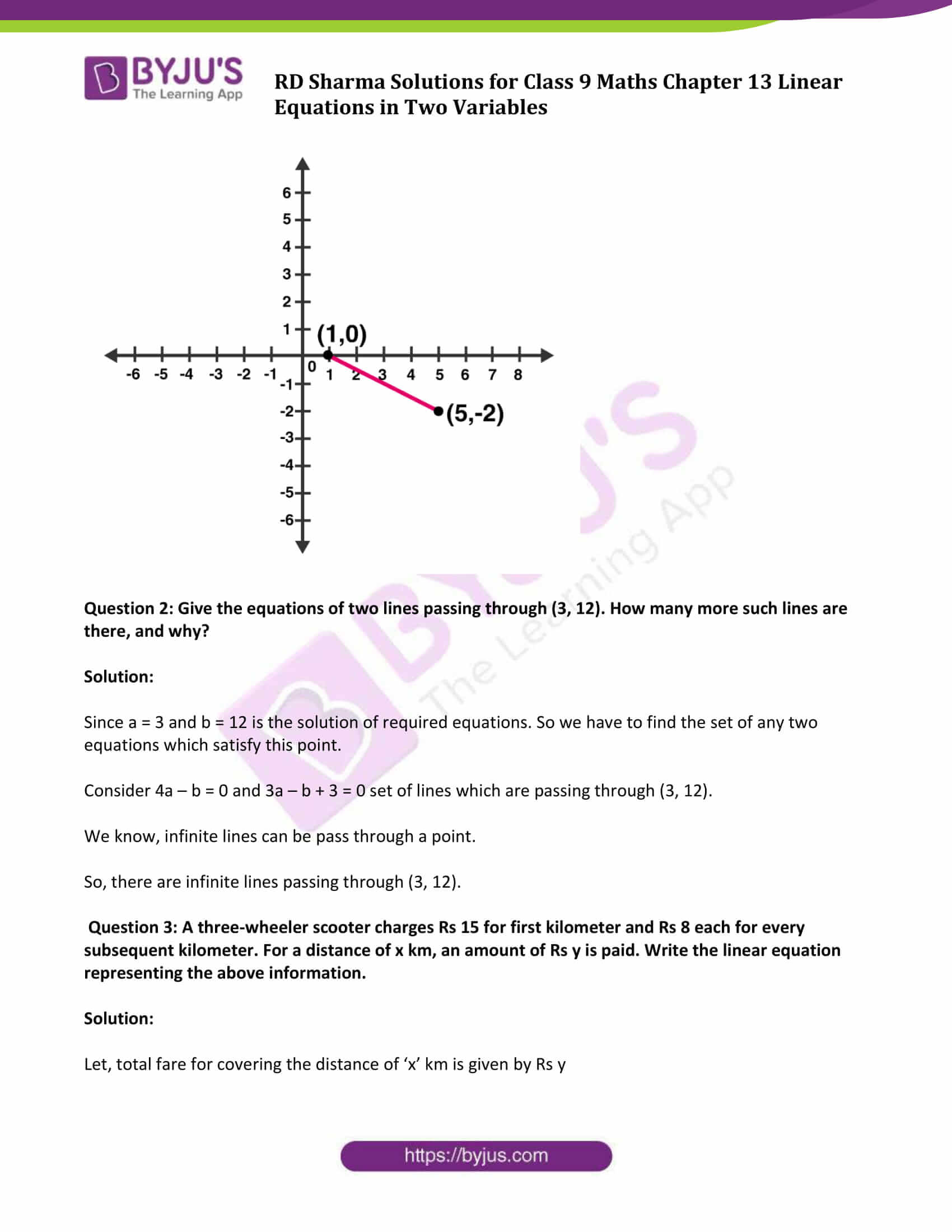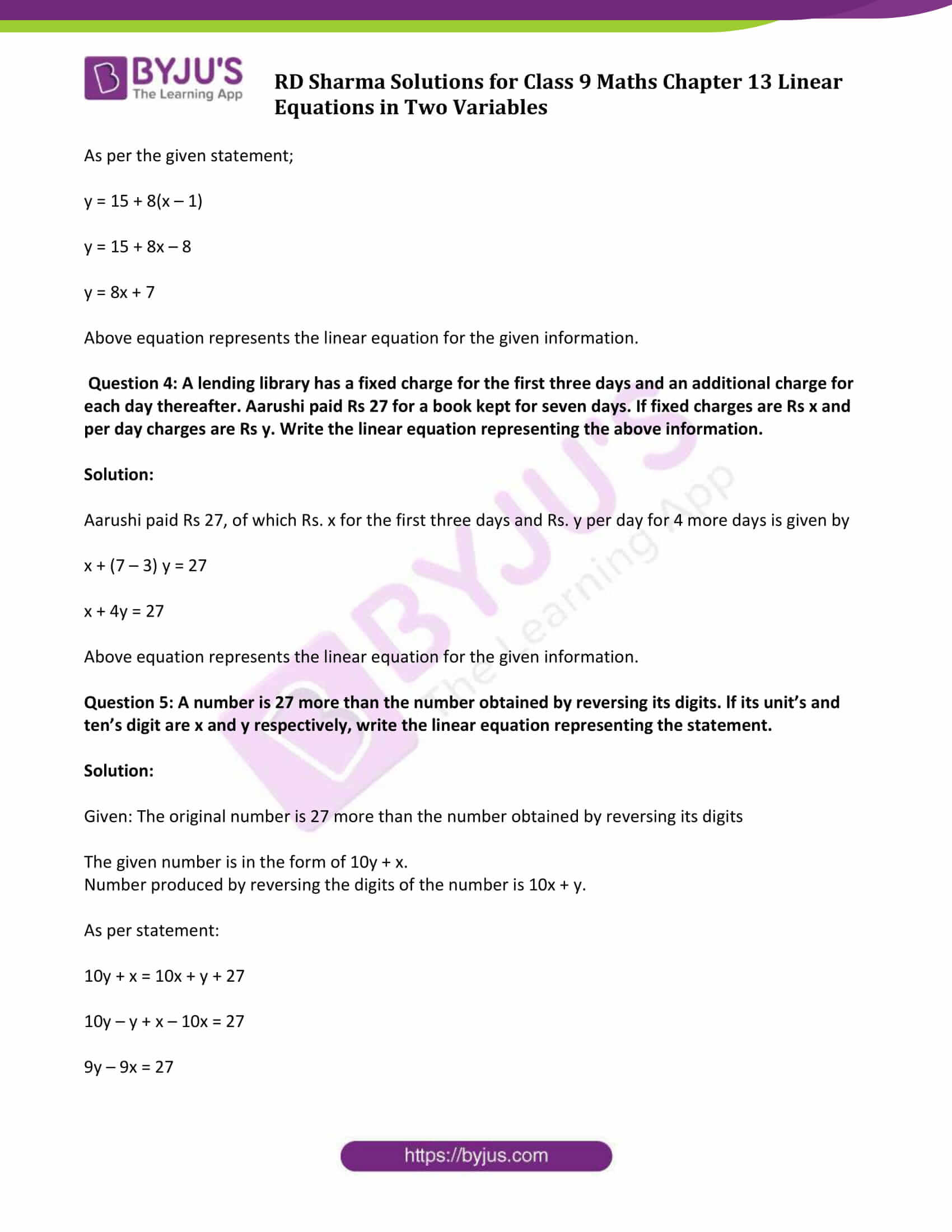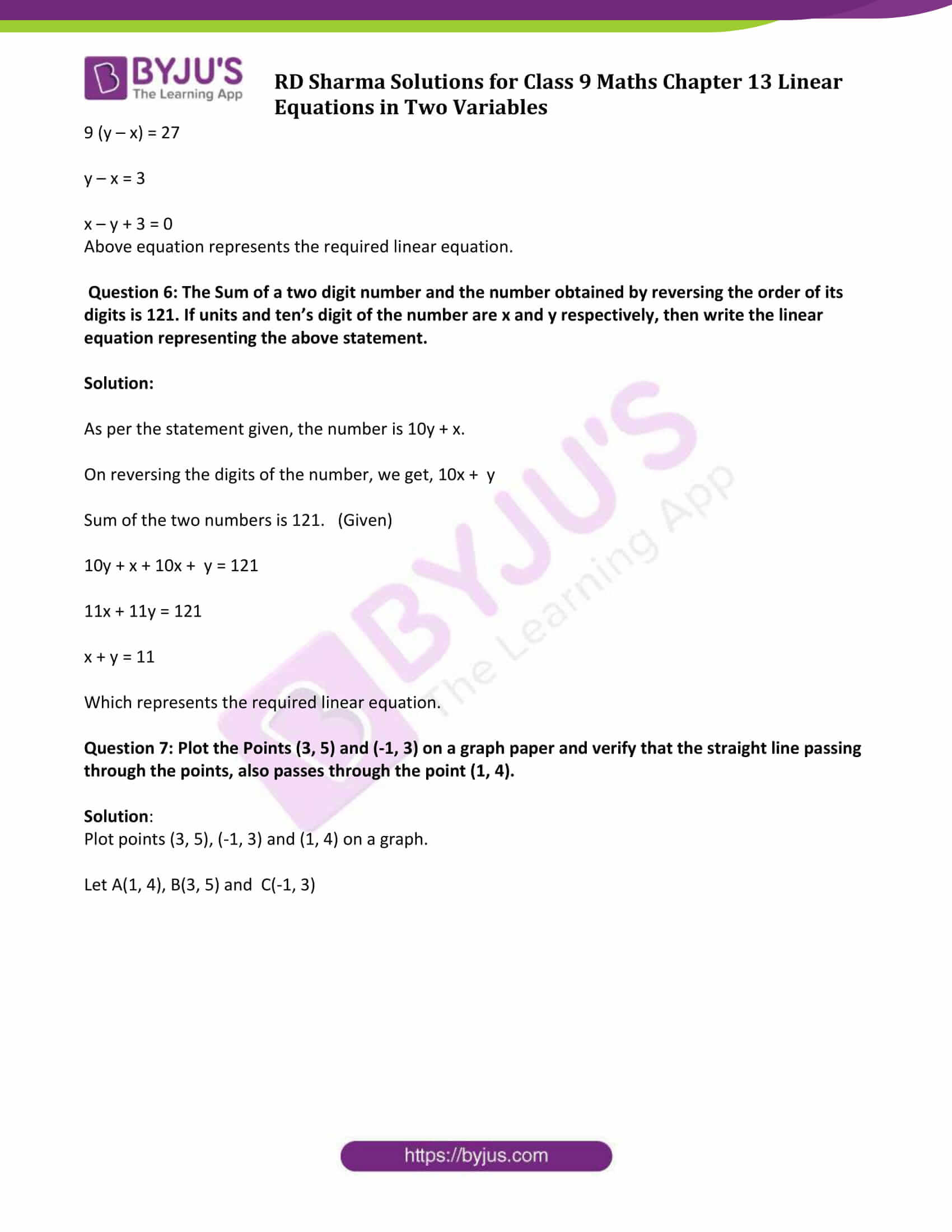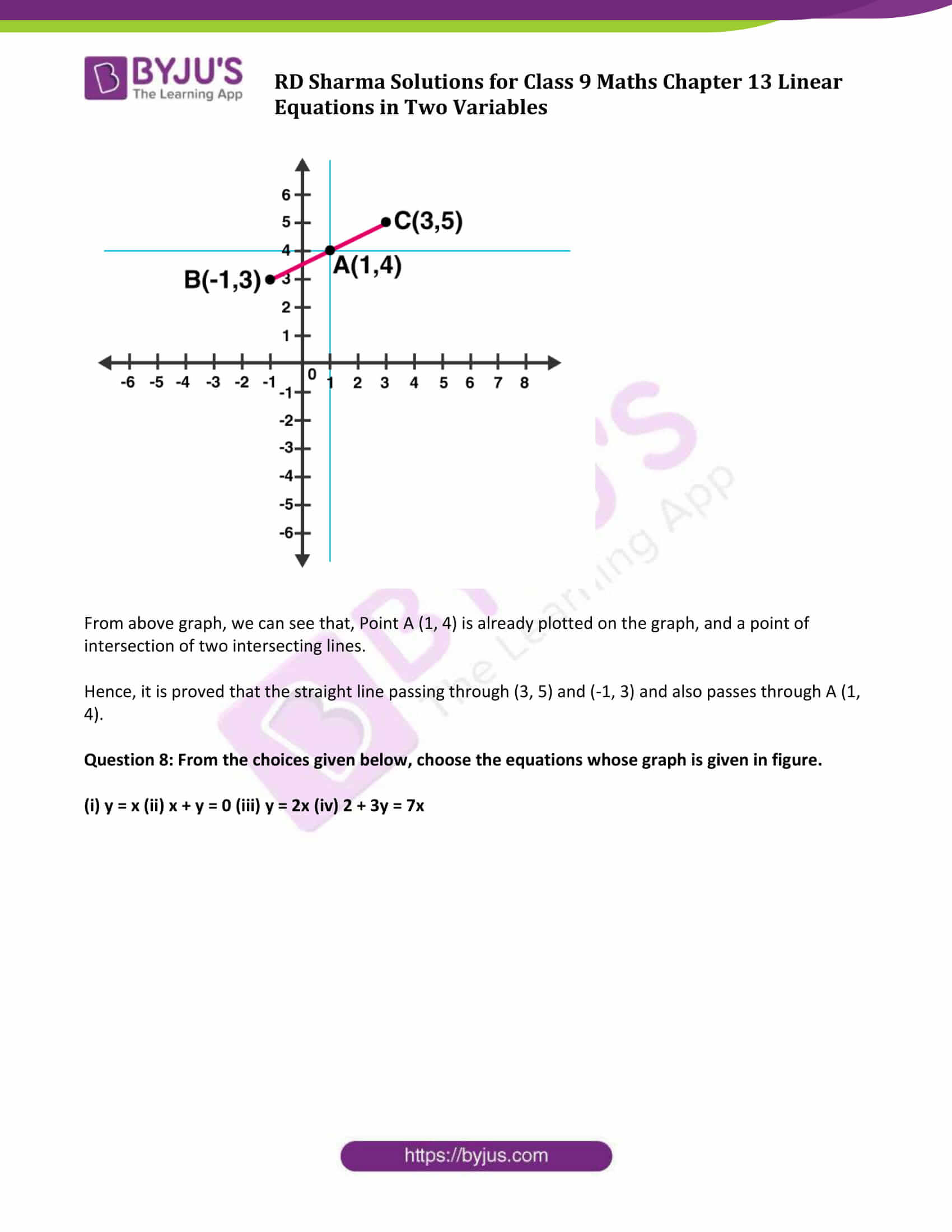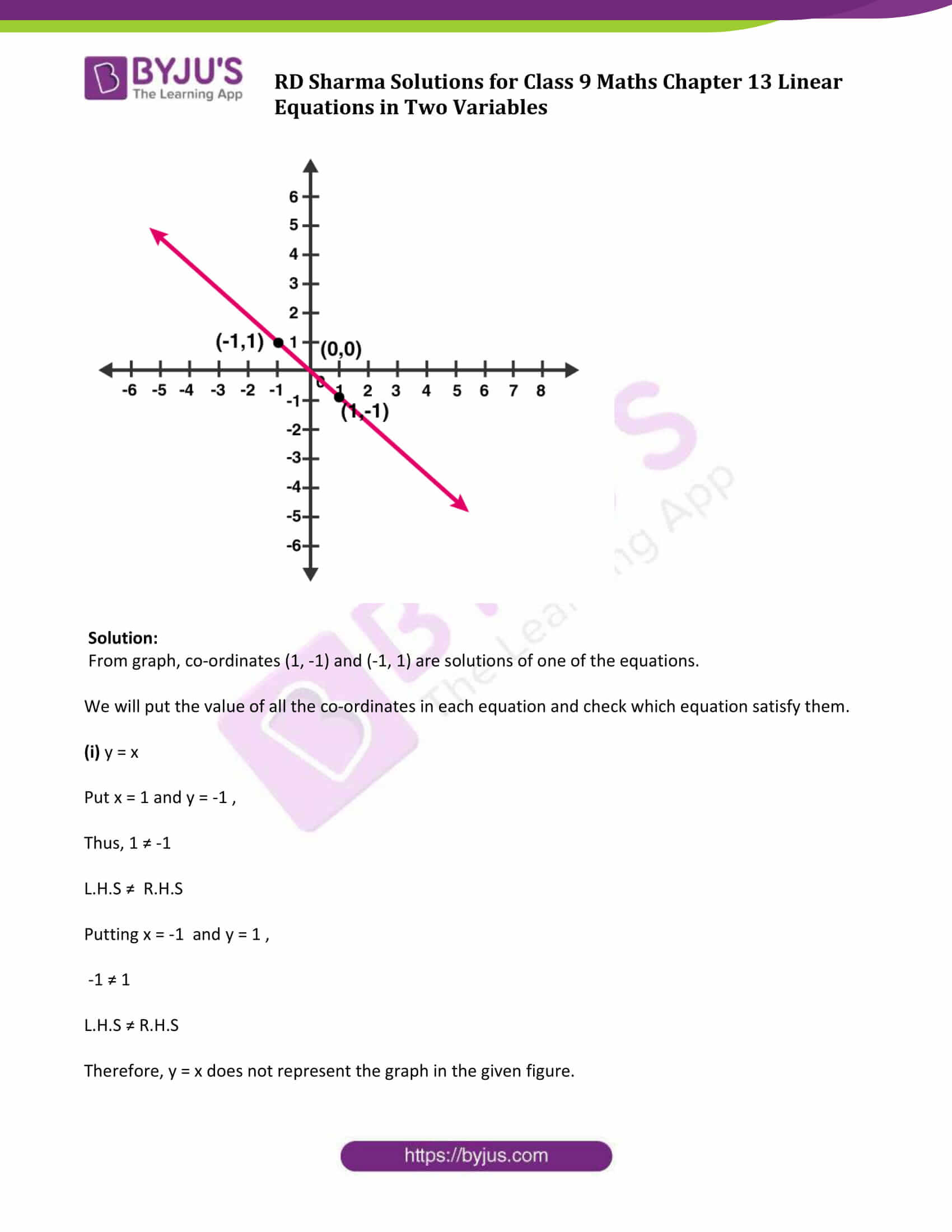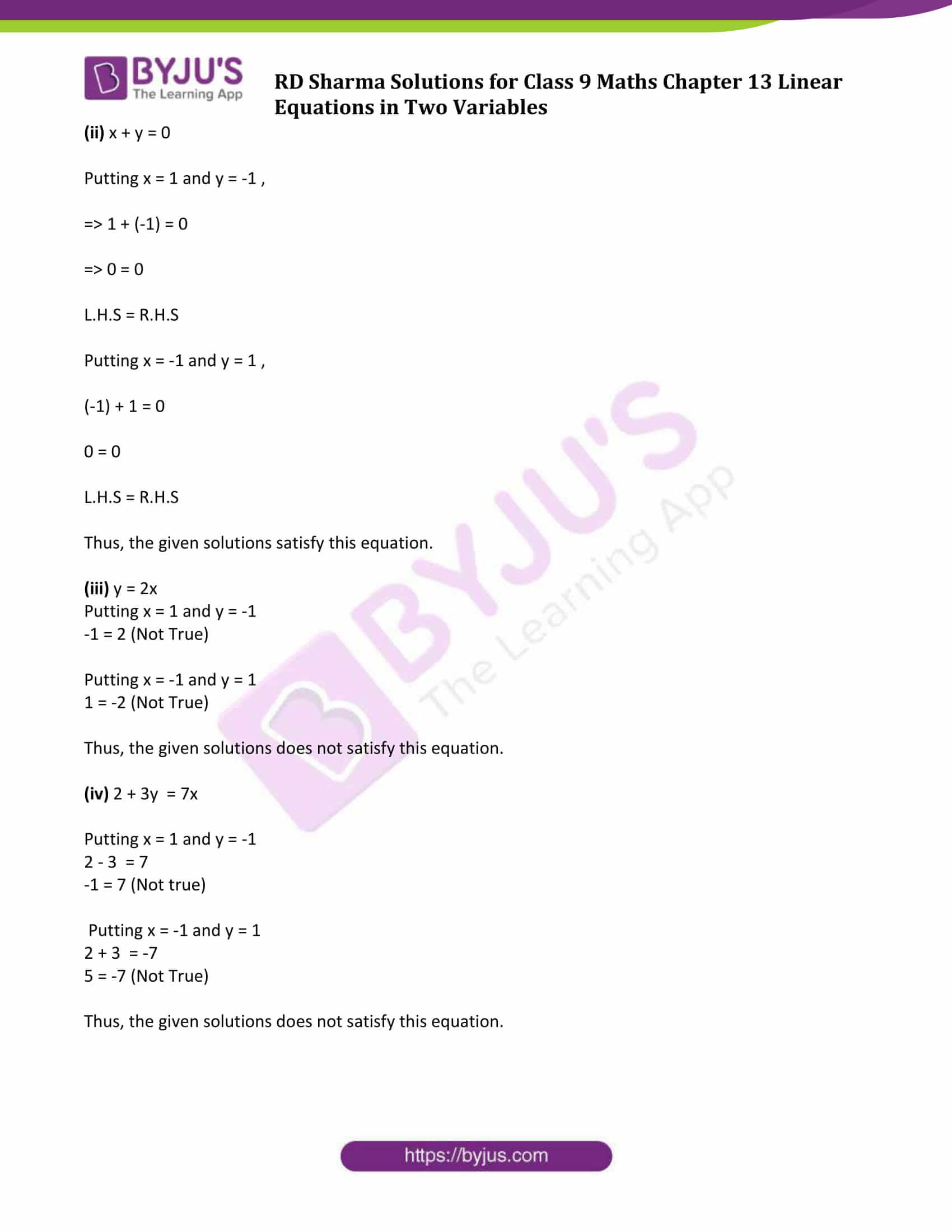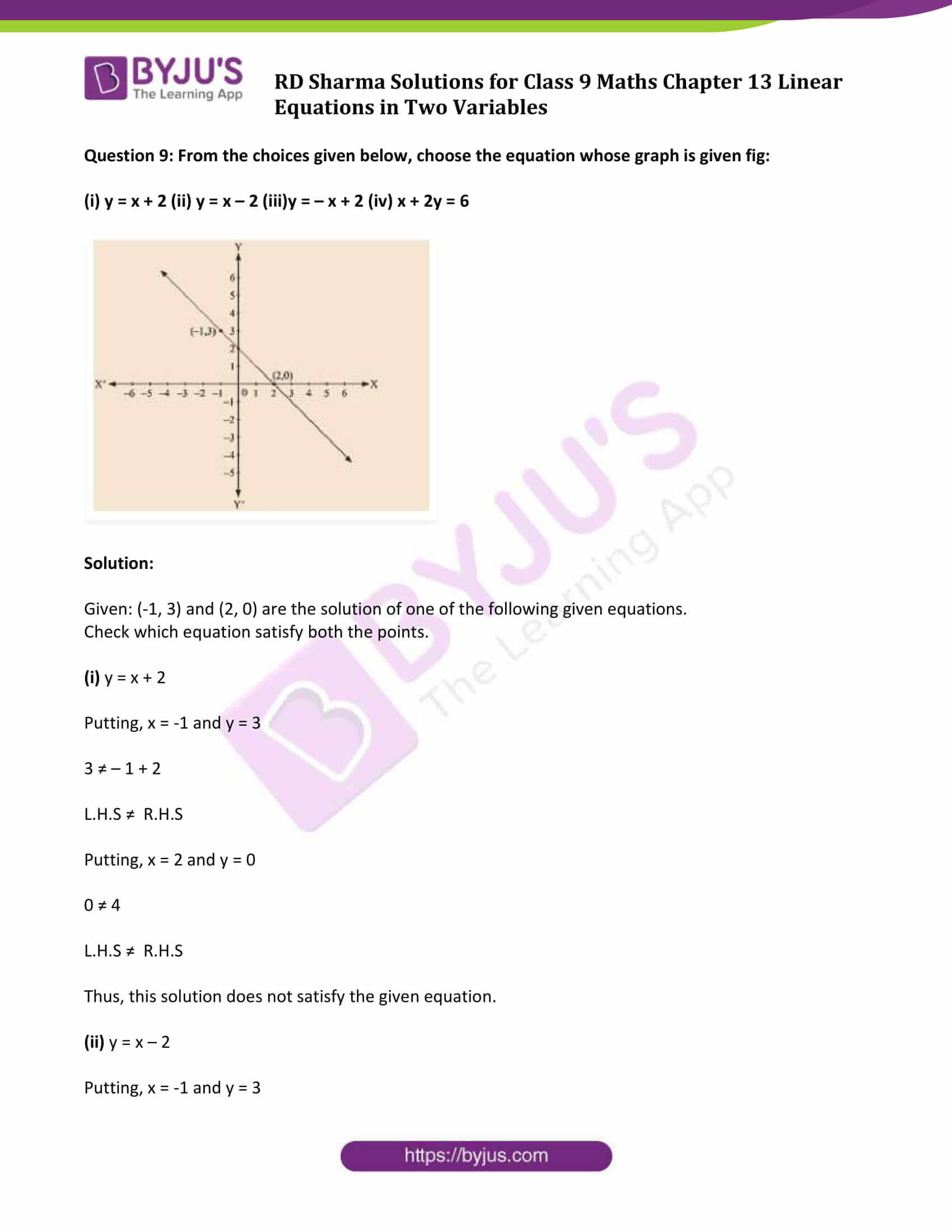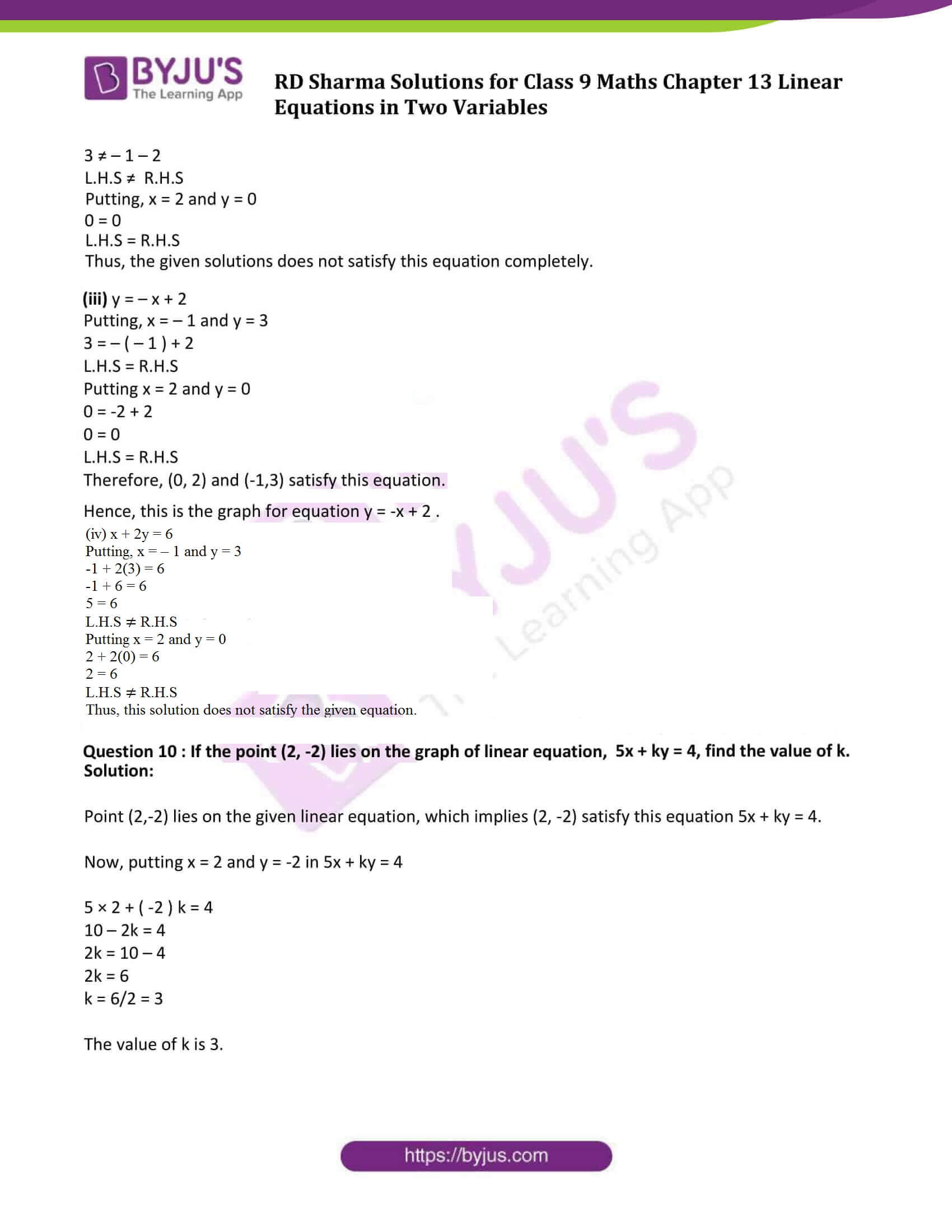### Access Answers to Maths RD Sharma Solutions for Class 9 Chapter 13 Linear Equations in Two Variables Exercise 13.3 Page number 13.23

Question 1: Draw the graph of each of the following linear equations in two variables:

(i) x + y = 4 (ii) x – y = 2 (iii) -x + y = 6

(iv) y = 2x (v) 3x + 5y = 15 (vi) x/2 − y/3 = 2

(vii) (x−2)/3 = y – 3 (viii) 2y = -x +1

Solution:

(i) Given : x + y = 4

or y = 4 – x,

Find values of x and y:

Putting x = 0 ⇒ y = 4

Putting x = 4 ⇒ y = 0

Graph:

Mark points (0, 4) and (4, 0) on the graph and join them.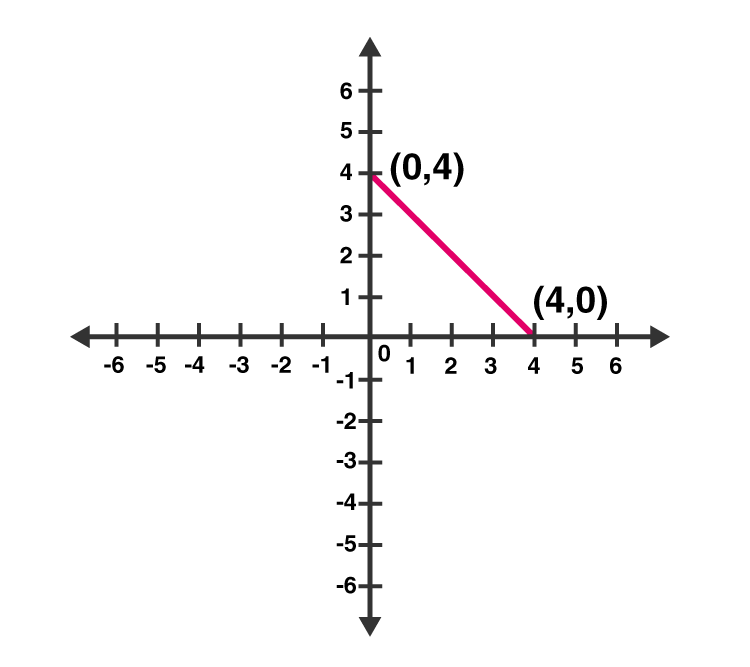(ii) Given: x – y = 2

So, y = x – 2

Putting x = 0 ⇒ y = – 2

Putting x = 2 ⇒ y = 0

Graph:

Mark points (0, -2) and (2, 0) on the graph and join them.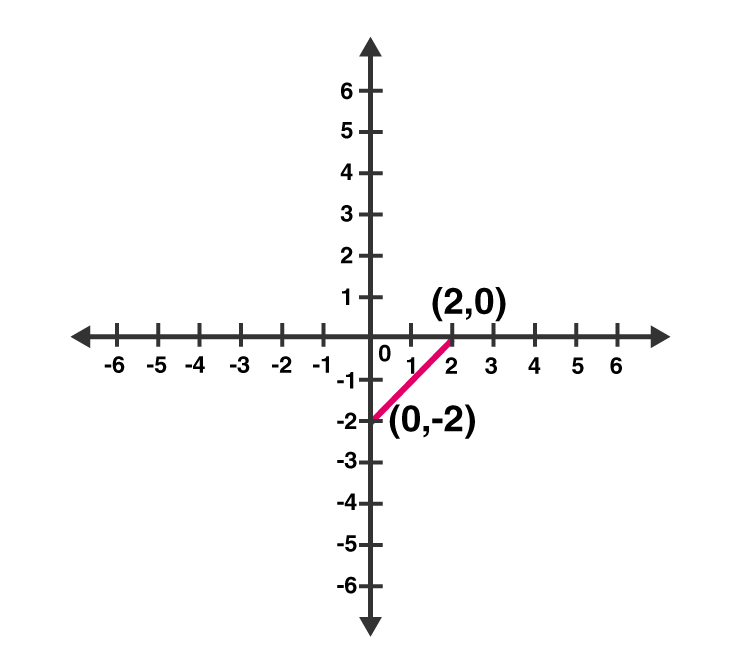(iii) Given: – x + y = 6

So, y = 6 + x

Putting x = 0 ⇒ y =6

Putting x = -6 ⇒ y = 0

Graph:

Mark points (0, 6) and (-6, 0) on the graph and join them.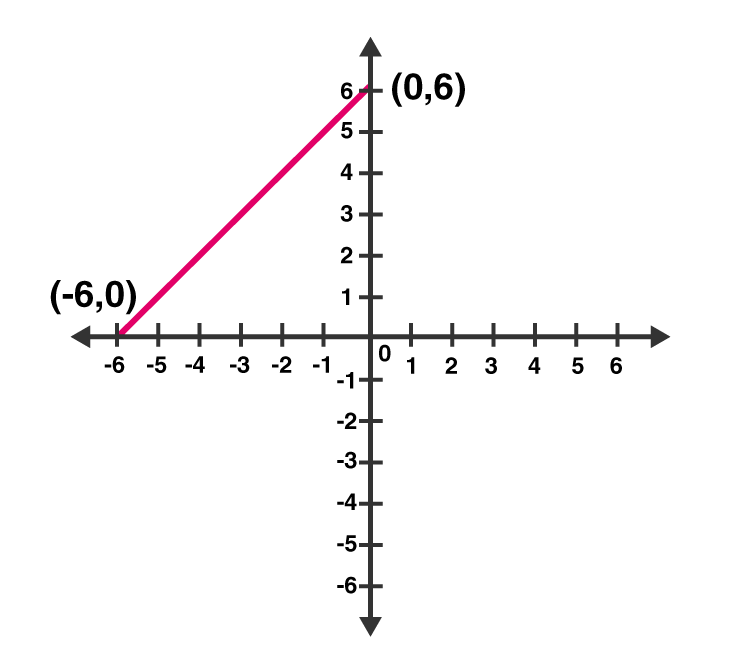(iv) Given: y = 2x

Put x = 1 ⇒ y = 2

Put x = 3 ⇒ y = 6

Graph:

Mark points (1, 2) and (3, 6) on the graph and join them.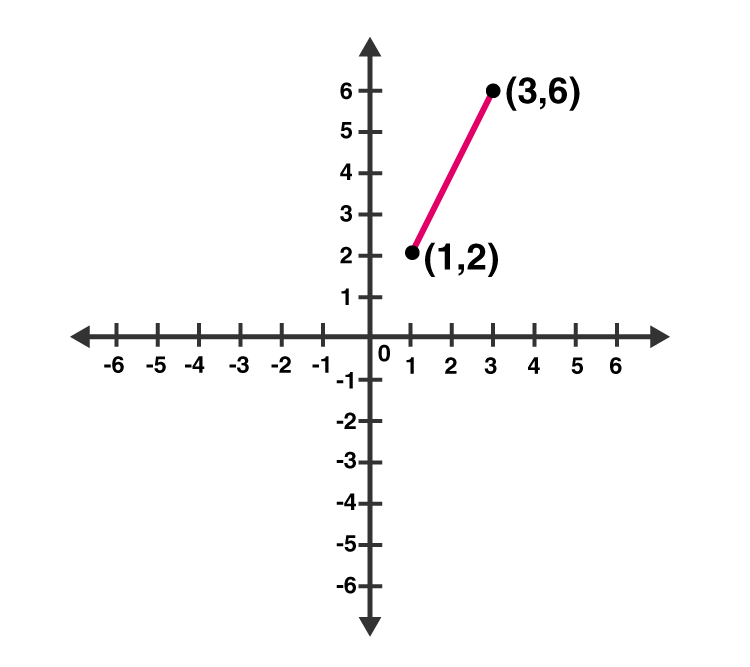(v) Given: 3x + 5y = 15

Or 5y = 15 – 3x

Putting x = 0 ⇒ 5y = 15 ⇒ y =3

Putting x = 5 ⇒ 5y = 0 ⇒ y = 0

Graph:

Mark points (0, 3) and (5, 0) on the graph and join them.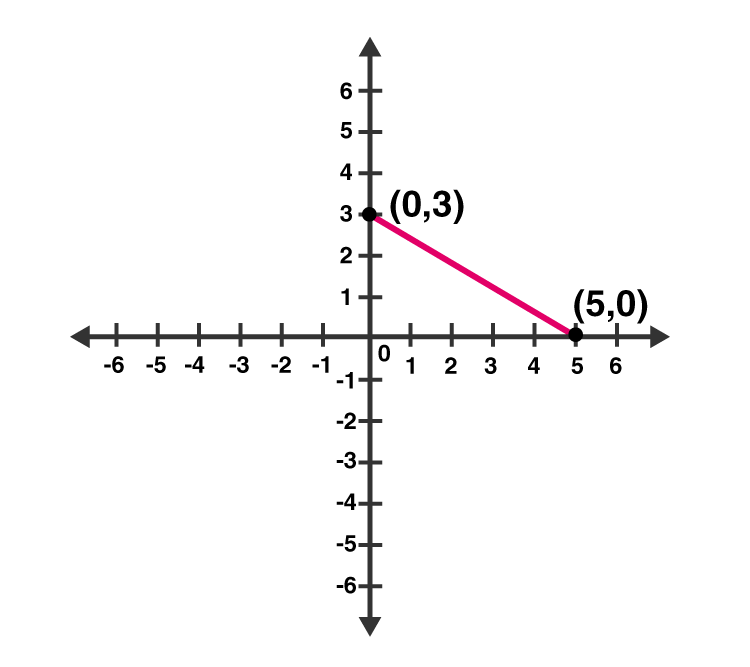(vi) Given: x/2 – y/3 = 2

3x – 2y = 12

y = (3x–12)/2

Putting x = 0 ⇒ y = -6

Putting x = 4 ⇒ y = 0

Graph:

Mark points (0, -6) and (4, 0) on the graph and join them.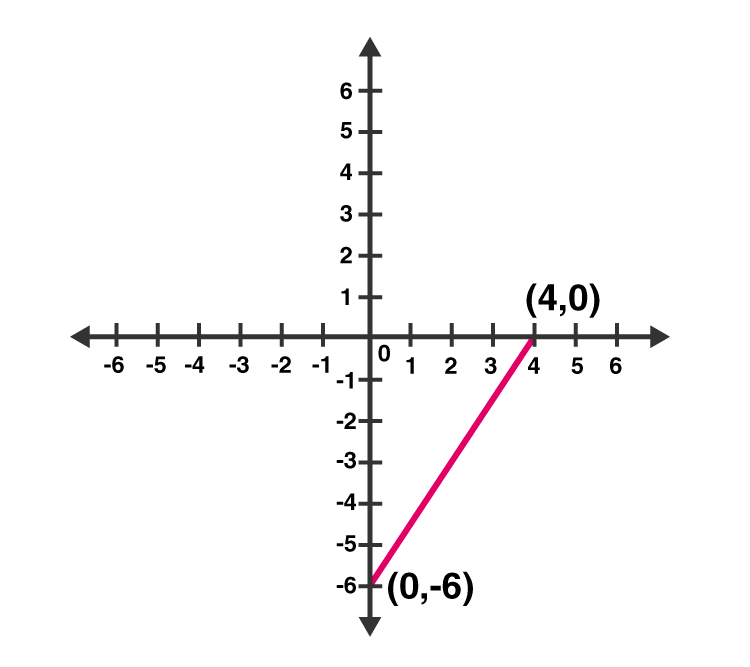(vii) Given: (x −2)/3 = y − 3

x – 2 = 3(y – 3)

x – 2 = 3y – 9

x = 3y – 7

Now, put x = 5 in x = 3y – 7

y = 4

Putting x = 8 in x = 3y – 7,

y = 5

Graph:

Mark points (5, 4) and (8, 5) on the graph and join them.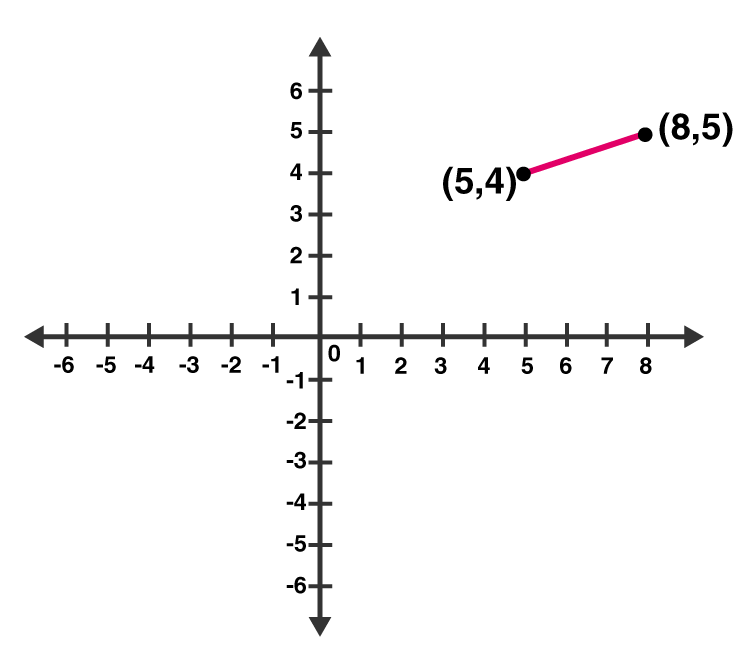(viii) Given: 2y = – x +1

2y = 1 – x

Now, putting x = 1 in 2y = 1 – x, we get;

y = 0

Again, putting x = 5 in 2y = 1 – x, we get;

y = -2

Graph:

Mark points (1, 0) and (5, -2) on the graph and join them.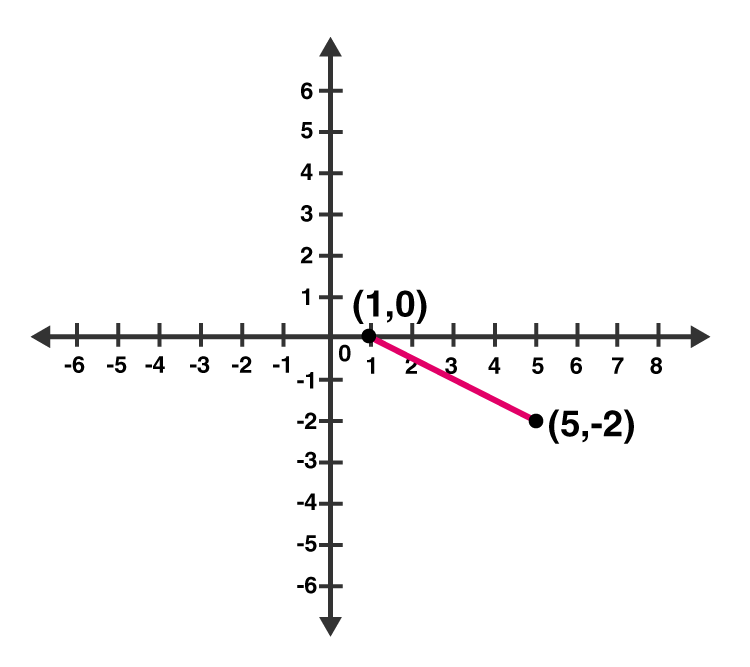Question 2: Give the equations of two lines passing through (3, 12). How many more such lines are there, and why?

Solution:

Since a = 3 and b = 12 is the solution of required equations. So we have to find the set of any two equations which satisfy this point.

Consider 4a – b = 0 and 3a – b + 3 = 0 set of lines which are passing through (3, 12).

We know, infinite lines can be pass through a point.

So, there are infinite lines passing through (3, 12).

Question 3: A three-wheeler scooter charges Rs 15 for first kilometer and Rs 8 each for every subsequent kilometer. For a distance of x km, an amount of Rs y is paid. Write the linear equation representing the above information.

Solution:

Let, total fare for covering the distance of ‘x’ km is given by Rs y

As per the given statement;

y = 15 + 8(x – 1)

y = 15 + 8x – 8

y = 8x + 7

Above equation represents the linear equation for the given information.

Question 4: A lending library has a fixed charge for the first three days and an additional charge for each day thereafter. Aarushi paid Rs 27 for a book kept for seven days. If fixed charges are Rs x and per day charges are Rs y. Write the linear equation representing the above information.

Solution:

Aarushi paid Rs 27, of which Rs. x for the first three days and Rs. y per day for 4 more days is given by

x + (7 – 3) y = 27

x + 4y = 27

Above equation represents the linear equation for the given information.

Question 5: A number is 27 more than the number obtained by reversing its digits. lf its unit’s and ten’s digit are x and y respectively, write the linear equation representing the statement.

Solution:

Given: The original number is 27 more than the number obtained by reversing its digits

The given number is in the form of 10y + x.

Number produced by reversing the digits of the number is 10x + y.

As per statement:

10y + x = 10x + y + 27

10y – y + x – 10x = 27

9y – 9x = 27

9 (y – x) = 27

y – x = 3

x – y + 3 = 0

Above equation represents the required linear equation.

Question 6: The Sum of a two digit number and the number obtained by reversing the order of its digits is 121. If units and ten’s digit of the number are x and y respectively, then write the linear equation representing the above statement.

Solution:

As per the statement given, the number is 10y + x.

On reversing the digits of the number, we get, 10x + y

Sum of the two numbers is 121. (Given)

10y + x + 10x + y = 121

11x + 11y = 121

x + y = 11

Which represents the required linear equation.

Question 7: Plot the Points (3, 5) and (-1, 3) on a graph paper and verify that the straight line passing through the points, also passes through the point (1, 4).

Solution:

Plot points (3, 5), (-1, 3) and (1, 4) on a graph.

Let A(1, 4), B(3, 5) and C(-1, 3)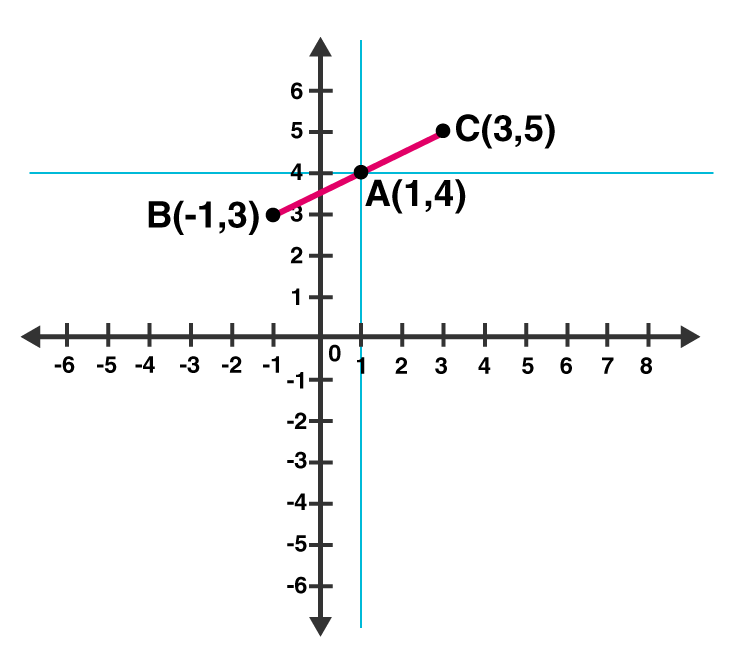From above graph, we can see that, Point A (1, 4) is already plotted on the graph, and a point of intersection of two intersecting lines.

Hence, it is proved that the straight line passing through (3, 5) and (-1, 3) and also passes through A (1, 4).

Question 8: From the choices given below, choose the equations whose graph is given in figure.

(i) y = x (ii) x + y = 0 (iii) y = 2x (iv) 2 + 3y = 7x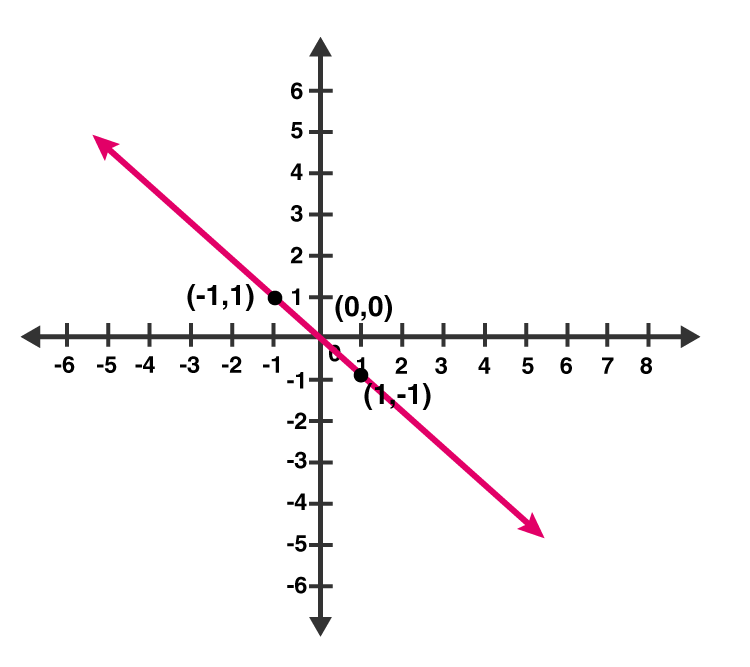Solution:

From graph, co-ordinates (1, -1) and (-1, 1) are solutions of one of the equations.

We will put the value of all the co-ordinates in each equation and check which equation satisfy them.

(i) y = x

Put x = 1 and y = -1 ,

Thus, 1 ≠ -1

L.H.S ≠ R.H.S

Putting x = -1 and y = 1 ,

-1 ≠ 1

L.H.S ≠ R.H.S

Therefore, y = x does not represent the graph in the given figure.

(ii) x + y = 0

Putting x = 1 and y = -1 ,

⇒ 1 + (-1) = 0

⇒ 0 = 0

L.H.S = R.H.S

Putting x = -1 and y = 1 ,

(-1) + 1 = 0

0 = 0

L.H.S = R.H.S

Thus, the given solutions satisfy this equation.

(iii) y = 2x

Putting x = 1 and y = -1

-1 = 2 (Not True)

Putting x = -1 and y = 1

1 = -2 (Not True)

Thus, the given solutions does not satisfy this equation.

(iv) 2 + 3y = 7x

Putting x = 1 and y = -1

2 – 3 = 7

-1 = 7 (Not true)

Putting x = -1 and y = 1

2 + 3 = -7

5 = -7 (Not True)

Thus, the given solutions does not satisfy this equation.

Question 9: From the choices given below, choose the equation whose graph is given fig:

(i) y = x + 2 (ii) y = x – 2 (iii)y = – x + 2 (iv) x + 2y = 6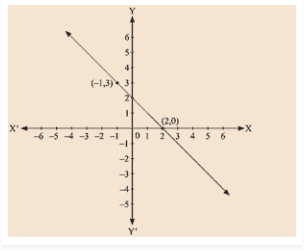Solution:

Given: (-1, 3) and (2, 0) are the solution of one of the following given equations.

Check which equation satisfy both the points.

(i) y = x + 2

Putting, x = -1 and y = 3

3 ≠ – 1 + 2

L.H.S ≠ R.H.S

Putting, x = 2 and y = 0

0 ≠ 4

L.H.S ≠ R.H.S

Thus, this solution does not satisfy the given equation.

(ii) y = x – 2

Putting, x = -1 and y = 3

3 ≠ – 1 – 2

L.H.S ≠ R.H.S

Putting, x = 2 and y = 0

0 = 0

L.H.S = R.H.S

Thus, the given solutions does not satisfy this equation completely.

(iii) y = – x + 2

Putting, x = – 1 and y = 3

3 = – ( – 1 ) + 2

L.H.S = R.H.S

Putting x = 2 and y = 0

0 = -2 + 2

0 = 0

L.H.S = R.H.S

Therefore, (0, 2) and (-1,3) satisfy this equation.

Hence, this is the graph for equation y = -x + 2 .

(iv) x + 2y = 6

Putting, x = – 1 and y = 3

-1 + 2(3) = 6

-1 + 6 = 6

5 = 6

L.H.S ≠ R.H.S

Putting x = 2 and y = 0

2 + 2(0) = 6

2 = 6

L.H.S ≠ R.H.S

Thus, this solution does not satisfy the given equation.

Question 10 : If the point (2, -2) lies on the graph of linear equation, 5x + ky = 4, find the value of k.

Solution:

Point (2,-2) lies on the given linear equation, which implies (2, -2) satisfy this equation 5x + ky = 4.

Now, putting x = 2 and y = -2 in 5x + ky = 4

5 × 2 + ( -2 ) k = 4

10 – 2k = 4

2k = 10 – 4

2k = 6

k = 6/2 = 3

The value of k is 3.

## RD Sharma Solutions for Class 9 Maths Chapter 13 Linear Equations in Two Variables Exercise 13.3

Class 9 Maths Chapter 13 Linear Equations in Two Variables Exercise 13.3 is based on finding a solution of linear equations in two variables using graphs. Students can download RD Sharma class 9 exercise 13.3 of chapter 13 solutions and practice to score good marks in the examinations.